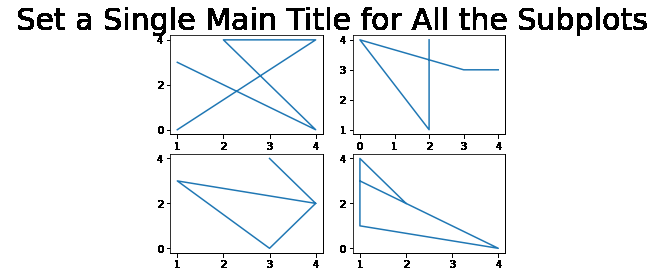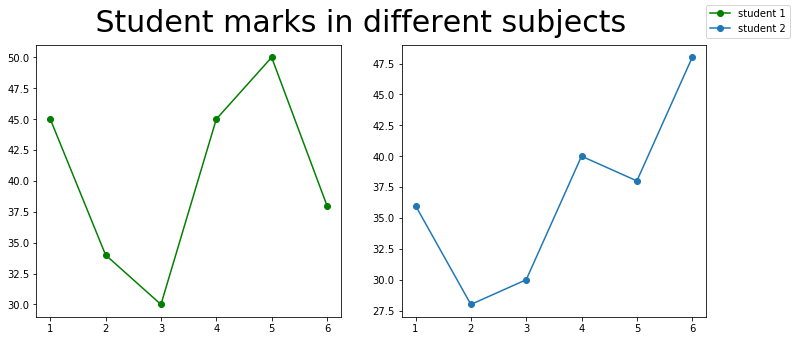# How to Set a Single Main Title for All the Subplots in Matplotlib?

A title in Matplotlib library describes the main subject of plotting the graphs. Setting a title for just one plot is easy using the title() method. By using this function only the individual title plots can be set but not a single title for all subplots. Hence, to set a single main title for all subplots, suptitle() method is used.

Syntax: suptitle(self, t, **kwargs)

Parameters: This method accept the following parameters that are discussed below:

• t : This parameter is the title text.
• x: This parameter is the x location of the text in figure coordinates.
• y: This parameter is the y location of the text in figure coordinates.
• horizontalalignment, ha : This parameter is the horizontal alignment of the text relative to (x, y).
• verticalalignment, va : This parameter is the vertical alignment of the text relative to (x, y).
• fontsize, size : This parameter is the font size of the text.
• fontweight, weight : This parameter is the font weight of the text.

Returns: This method returns the Text instance of the title.

## Setting a Single Title for All the Subplots

### Example 1:

In this example, we will import the required library and create a 2*2 plot. We are creating random data by using random.randint to plot our graph and then setting a single title for all the subplots.

## Python3

 `# importing packages` `import` `matplotlib.pyplot as plt` `import` `numpy as np`   `# making subplots objects` `fig, ax ``=` `plt.subplots(``2``, ``2``)`   `# draw graph` `ax[``0``][``0``].plot(np.random.randint(``0``, ``5``, ``5``), np.random.randint(``0``, ``5``, ``5``))` `ax[``0``][``1``].plot(np.random.randint(``0``, ``5``, ``5``), np.random.randint(``0``, ``5``, ``5``))` `ax[``1``][``0``].plot(np.random.randint(``0``, ``5``, ``5``), np.random.randint(``0``, ``5``, ``5``))` `ax[``1``][``1``].plot(np.random.randint(``0``, ``5``, ``5``), np.random.randint(``0``, ``5``, ``5``))`     `fig.suptitle(``' Set a Single Main Title for All the Subplots '``, fontsize``=``30``)`   `plt.show()`

Output:### Example 2:

Here, we are creating data to plot our graph and using a marker.

## Python3

 `import` `matplotlib.pyplot as plt` `import` `numpy as np`   `fig, (ax1, ax2) ``=` `plt.subplots(``1``, ``2``, figsize``=``(``12``, ``5``))`   `x1 ``=` `[``1``, ``2``, ``3``, ``4``, ``5``, ``6``]` `y1 ``=` `[``45``, ``34``, ``30``, ``45``, ``50``, ``38``]` `y2 ``=` `[``36``, ``28``, ``30``, ``40``, ``38``, ``48``]`   `labels ``=` `[``"student 1"``, ``"student 2"``]`   `# Add title to subplot` `fig.suptitle(``' Student marks in different subjects '``, fontsize``=``30``)`   `# Creating the sub-plots.` `l1 ``=` `ax1.plot(x1, y1, ``'o-'``, color``=``'g'``)` `l2 ``=` `ax2.plot(x1, y2, ``'o-'``)`   `fig.legend([l1, l2], labels``=``labels,` `           ``loc``=``"upper right"``)` `plt.subplots_adjust(right``=``0.9``)`   `plt.show()`

Output:Whether you're preparing for your first job interview or aiming to upskill in this ever-evolving tech landscape, GeeksforGeeks Courses are your key to success. We provide top-quality content at affordable prices, all geared towards accelerating your growth in a time-bound manner. Join the millions we've already empowered, and we're here to do the same for you. Don't miss out - check it out now!

Previous
Next